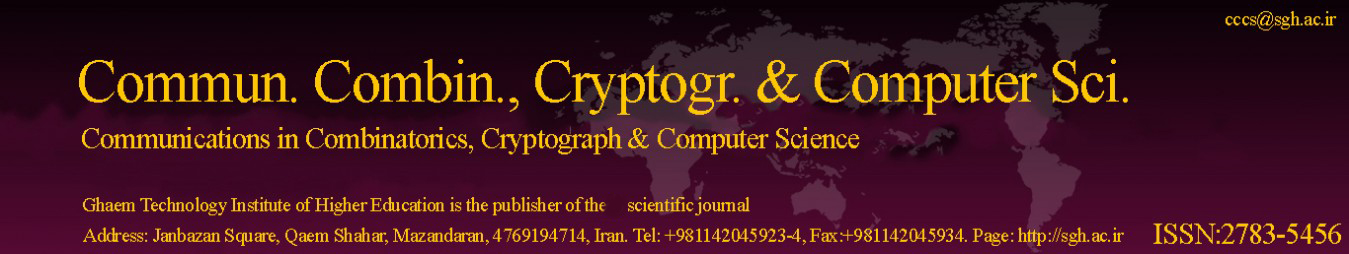Original Research Article

Article volume = 2022 and issue = 2

Pages: 94–104

Article publication Date: November 21, 2022

# A Public-Key Cryptographic Model Based on Hybrid Key Exchange Technique using Affine-Hill Cipher

#### Kshitish Kumar Mohanta and Deena Sunil Sharanappa

Department of Mathematics, Indira Gandhi National Tribal University, Amarkantak, 484887, Anuppur, Madhya Pradesh, India.

##### Abstract:

In this paper, a hybrid method for creating key matrices using the multi-key exchange algorithm was suggested. It combines the ElGamal and Elliptic Curve Diffe Hellman (ECDH) algorithms. The key matrix for encryption and decryption uses the generalized Fibonacci matrix modulo p and the inverse of the generalized Fibonacci matrix modulo p. Generalized Fibonacci sequences are used to construct these matrices. With the help of the proposed combined algorithm, we only need to sent the order and power of the generalized Fibonacci matrix and the seeds of the generalized Fibonacci sequence, rather than the entire key matrix. The Affine Hill Cipher technique is used in this public key cryptography model to encrypt plain text and decrypt cipher text. The proposed method increases security strength of the model due to use of four secrete integers a,b,(n,m).

##### Keywords:

Public key cryptography, Elliptic Curve Diffie-Hellman(ECDH) Algorithm, ElGamal Algorithm, Generalized Fibonacci matrix, Affine-Hill Cipher.

##### References:
•  B. Prasad, FIBONACCI MATRICES AND HYBRID MATRIX CRYPTOGRAPHY, Discrete Mathematics, Algorithms and Applications, 6(1) (2014), 1450009. 1
•  C. Paar, J. Pelzl, Understanding cryptography: a textbook for students and practitioners, Springer Science & Business Media, 2009. 1, 3.2, 4
•  D.R. Stinson, Cryptography: theory and practice, Chapman and Hall/CRC, 2005. 1
•  G.Y. Lee, J.S. Kim, S.G. Lee, Factorizations and eigenvalues of fibonacci and symmetric fibonacci matrices, Fibonacci Quarterly, 40 (2002), pp. 203–211. 2
•  K. Prasad, H. Mahato, Cryptography using generalized fibonacci matrices with affine hill cipher, Journal of Discrete Mathematical Sciences and Cryptography, (2021), pp. 1–12. 1
•  K. Prasad, H. Mahato, M. Kumari, A novel public key cryptography based on generalized Lucas matrices, arXiv preprint arXiv:2202.08156, (2022). 1
•  L.S. Hill, Cryptography in an algebraic alphabet, The American Mathematical Monthly, 36 (1929), pp. 306–312. 4
•  M. Kumari, J. Tanti, On the role of the Fibonacci matrix as key in modified ECC, arXiv preprint arXiv:2112.11013, (2021). 1
•  M. Viswanath, M.R. Kumar, A public key cryptosystem using hiil’s cipher, Journal of Discrete Mathematical Sciences and Cryptography, 18 (2015), pp. 129–138. 1
•  N. Mehibel, M. Hamadouche, A new approach of elliptic curve Diffie-Hellman key exchange, in 2017 5th International Conference on Electrical Engineering-Boumerdes (ICEE-B). IEEE, 2017, pp. 1–6. 3.2
•  P. Stanimirovi´c, J. Nikolov, I. Stanimirovi´c, A generalization of fibonacci and lucas matrices, Discrete applied mathematics, 156 (2008), pp. 2606–2619. 2.1, 2.1, 2.2
•  P. Sundarayya, G. Vara Prasad, A public key cryptosystem using affine hill cipher under modulation of prime number, Journal of Information and Optimization Sciences, 40 (2019), pp. 919–930. 1
•  P. K. Ray, G. K. Dila, B. K. Patel, Application of some recurrence relations to cryptography using finite state machine, International Journal of Computer Science and Electronics Engineering(IJCSEE), 2(4) (2014). 1
•  S. Swain, C. Pratihary, P.K. Ray, Balancing and Lucas-Balancing Numbers and Their Application to Cryptography, Computer Engineering and Applications Journal, 5(1) (2016), pp.29-36. 1
•  T. ElGamal, A public key cryptosystem and a signature scheme based on discrete logarithms, IEEE transactions on information theory, 31 (1985), pp. 469–472. 1, 3.1
•  T. Koshy, Fibonacci and lucas numbers with applications, John Wiley & Sons, 2019. 1, 2
•  W. Diffie, M. Hellman, New directions in cryptography, IEEE transactions on Information Theory, 22 (1976), pp. 644–654. 3.1
•  Z. Zhang, Y. Zhang, The lucas matrix and some combinatorial identities, Indian Journal of Pure and Applied Mathematics, 38 (2007), p. 457. 2.1, 2